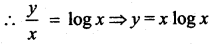Samacheer Kalvi 12th Maths Solutions Chapter 10 Ordinary Differential Equations Ex 10.6

Tamilnadu Samacheer Kalvi 12th Maths Solutions Chapter 10 Ordinary Differential Equations Ex 10.6

Solve the following differential equations:

Question 1.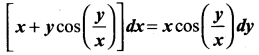Solution: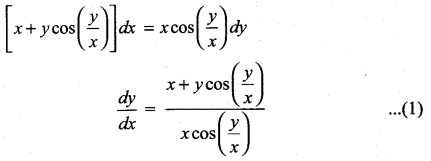This is a Homogeneous differential equation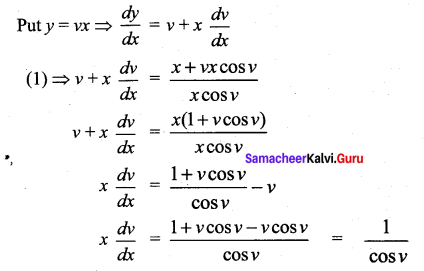Seperating the variables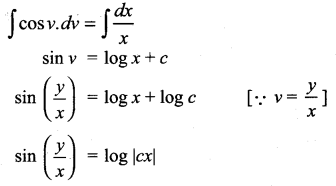Question 2.
(x3 + y3) dy – x2y dx = 0
Solution:
(x3 + y3) dy – x2y dx = 0
(x3 + y3) dy = x3y dx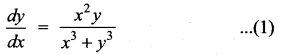This is a Homogeneous differential equation.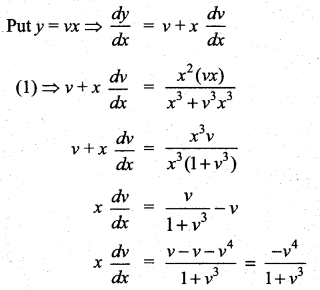Separating the variables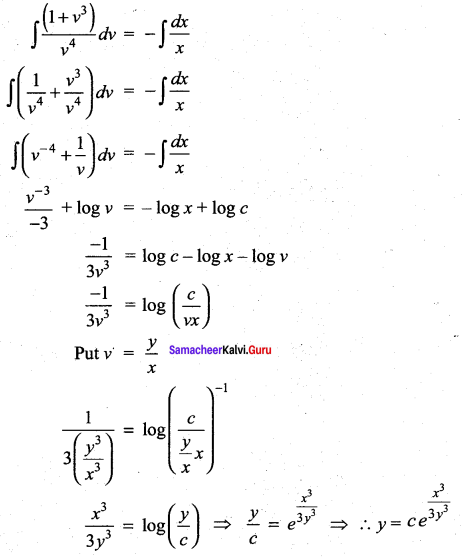Question 3.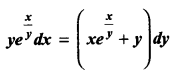Solution: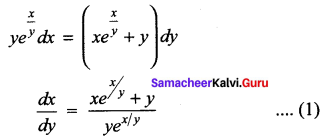This is a Homogeneous differential equation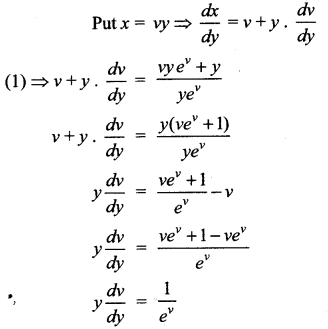Seperating the variables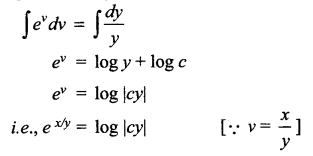Question 4.
2xydx + (x2 + 2y2) dy = 0
Solution:
2xy dx + (x2 + 2y2) dy = 0
(x2 + 2y2) dy = – 2xy dx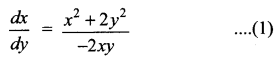This is a Homogeneous differential equation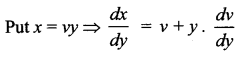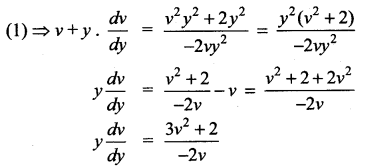Separating the variables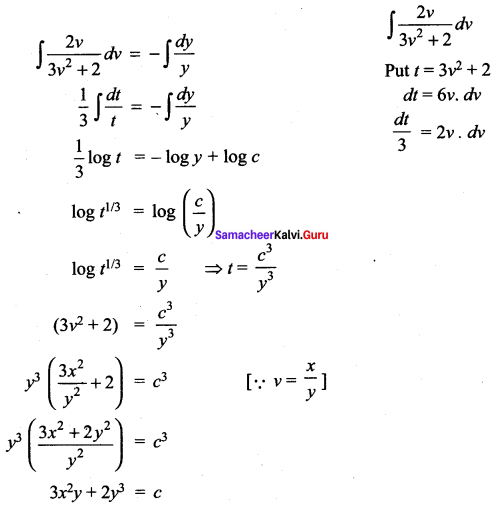Question 5.
(y2 – 2xy)dx = (x2 – 2xy)dy
Solution:
(y2 – 2xy) dx = (x2 – 2xy) dy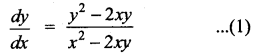This is a Homogeneous differential equation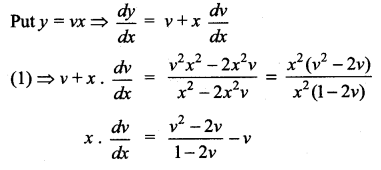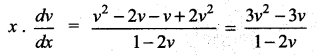Seperating the variables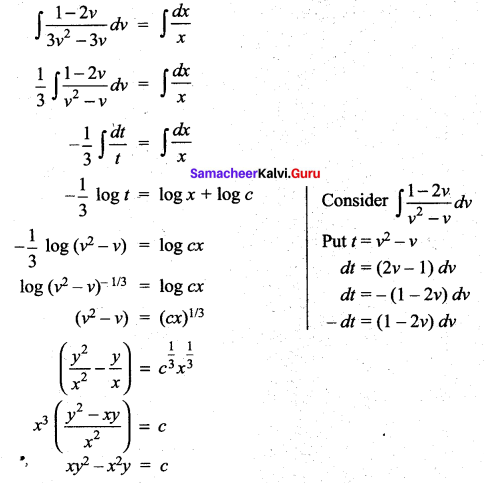Question 6.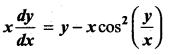Solution: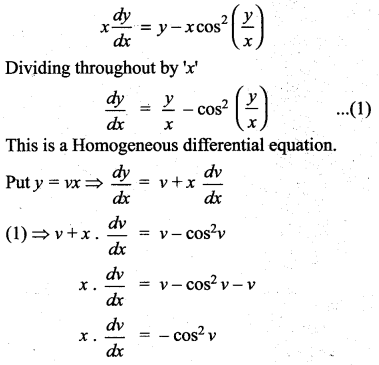Seperating the variables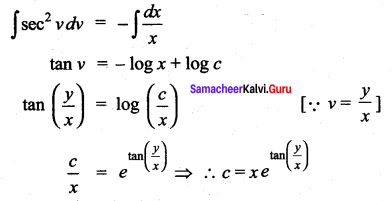Question 7.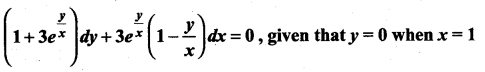Solution: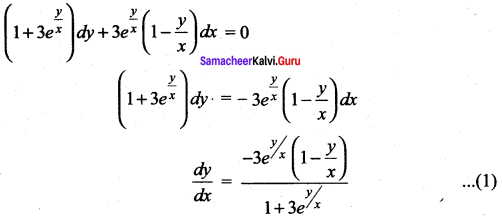This is a Homogeneous differential equation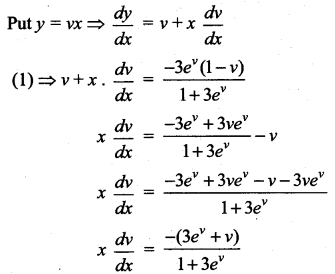Separating the variables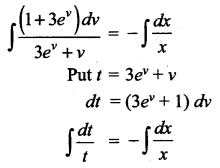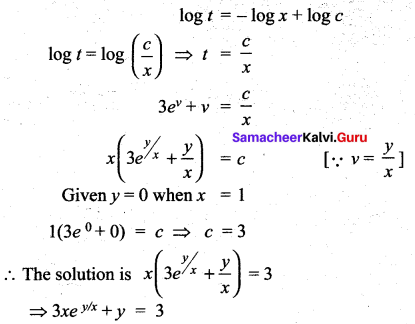Question 8.
(x2 + y2) dy = xy dx. It is given that y(1) = 1 and y(x0) = e. Find the value of x0.
Solution: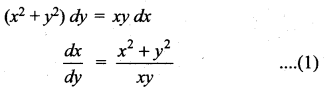This is a Homogeneous differential equation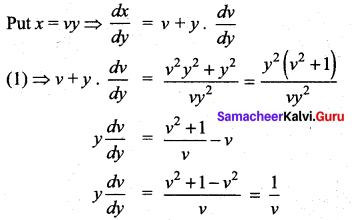Seperating the variables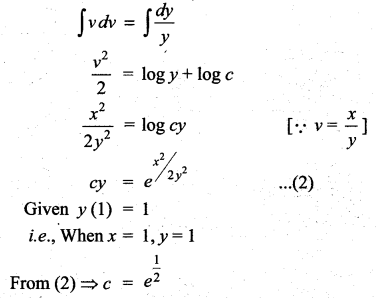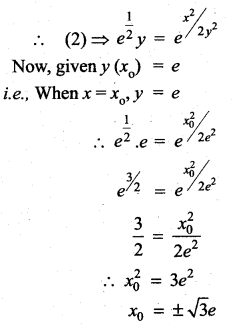Samacheer Kalvi 12th Maths Solutions Chapter 10 Ordinary Differential Equations Ex 10.6 Additional problems

Question 1.
Solve: (2$$\sqrt{x y}$$)dy + y dx = 0
Solution: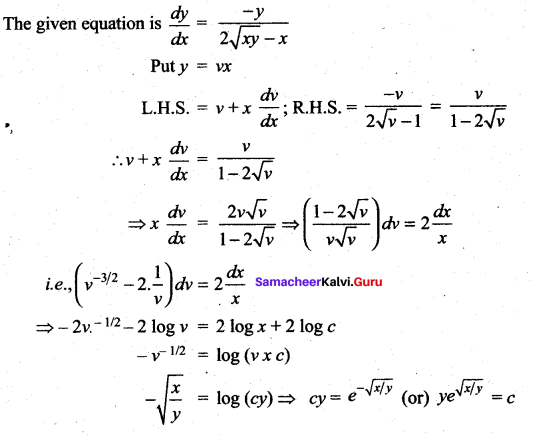Question 2.
Solve: (x3 + 3xy2)dx + (y3 + 3x2y)dy = 0
Solution: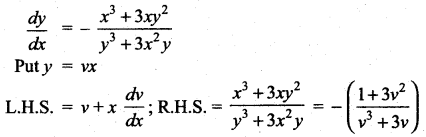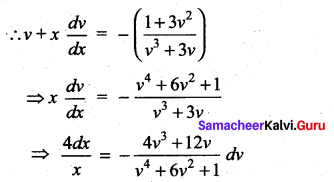Integrating, we have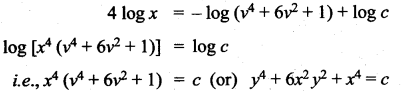Question 3.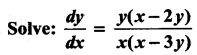Solution: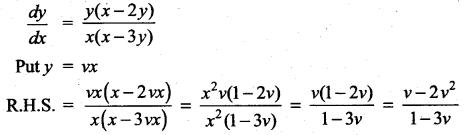Which is in terms of v alone.
⇒ the given problem comes under homogeneous type.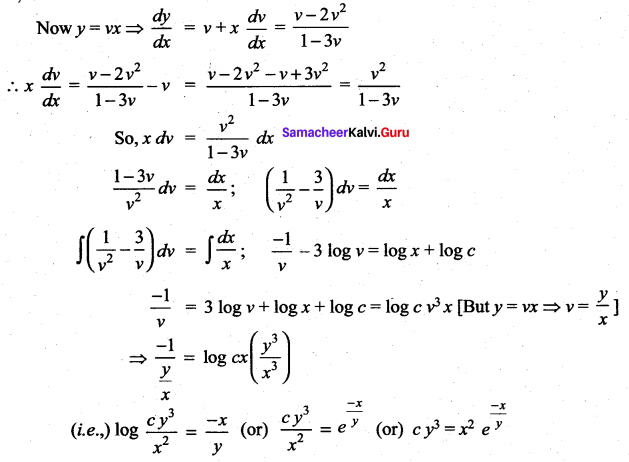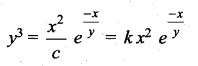Question 4.
Solve: (x2 + y2)dy = xy dx
Solution: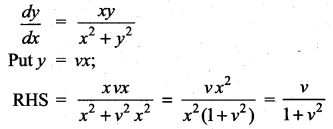Which is a function in v alone.
⇒ the given problem comes under homogeneous type.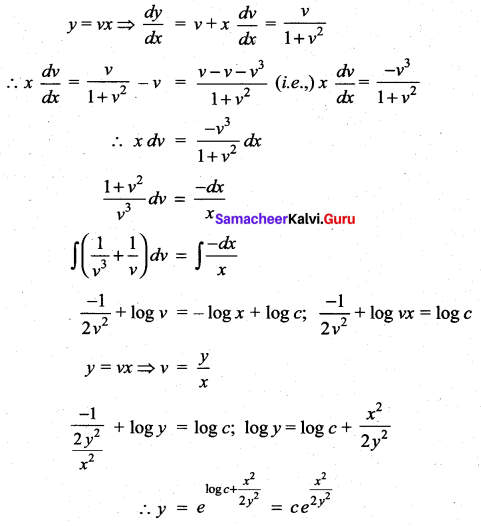Question 5.
Find the equation of the curve passing through (1, 0) and which has slope $$1+\frac{y}{x}$$ at (x, y).
Solution: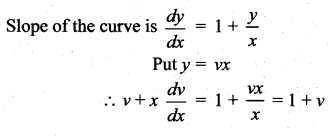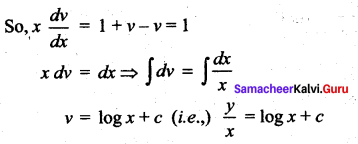Given the curve passes through (1, 0) ⇒ at x = 1, y = 0
0 = log 1 +c ⇒ c = 0